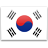L Q S T

Less Results
L Q S T
L Q S T
L Q S T
L Q S T
L Q S T
L Q S T
L Q S T
L Q S T
L Q S T
L Q S T
L Q S T
L Q S T
L Q S T
L Q S T
L Q S T

Less Results
L Q S T
L Q S
L Q S T
L Q S T
L Q S T